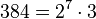# Groups of order 384

## Contents

See pages on algebraic structures of order 384| See pages on groups of a particular order

Information type Page summarizing information for groups of order 384
element structure (element orders, conjugacy classes, etc.) element structure of groups of order 384
subgroup structure subgroup structure of groups of order 384
linear representation theory linear representation theory of groups of order 384
projective representation theory of groups of order 384
modular representation theory of groups of order 384
endomorphism structure, automorphism structure endomorphism structure of groups of order 384
group cohomology group cohomology of groups of order 384

## Statistics at a glance

The number 384 has prime factorization$384 = 2^7 \cdot 3$. There are only two prime factors of this number. Order has only two prime factors implies solvable (by Burnside's$p^aq^b$-theorem) and hence all groups of this order are solvable groups (specifically, finite solvable groups). Another way of putting this is that the order is a solvability-forcing number. In particular, there is no simple non-abelian group of this order.

Quantity Value Explanation
Number of groups up to isomorphism 20169
Number of abelian groups up to isomorphism 15 (number of abelian groups of order$2^7$) times (number of abelian groups of order$3^1$) = (number of unordered integer partitions of 7) times (number of unordered integer partitions of 1) =$15 \times 1 = 15$
Number of nilpotent groups up to isomorphism 2328 (number of groups of order 128) times (number of groups of order 3) =$2328 \times 1 = 2328$
Number of solvable groups up to isomorphism 20169 There are only two prime factors of this number. Order has only two prime factors implies solvable (by Burnside's$p^aq^b$-theorem) and hence all groups of this order are solvable groups (specifically, finite solvable groups). Another way of putting this is that the order is a solvability-forcing number. In particular, there is no simple non-abelian group of this order.
Number of simple groups up to isomorphism 0 Follows from all groups of this order being solvable.

## GAP implementation

The order 384 is part of GAP's SmallGroup library. Hence, any group of order 384 can be constructed using the SmallGroup function by specifying its group ID. Also, IdGroup is available, so the group ID of any group of this order can be queried.

Further, the collection of all groups of order 384 can be accessed as a list using GAP's AllSmallGroups function. However, the list size may be too large relative to the memory allocation given in typical GAP installations. To overcome this problem, use the IdsOfAllSmallGroups function which stores and manipulates only the group IDs, not the groups themselves.

Here is GAP's summary information about how it stores groups of this order, accessed using GAP's SmallGroupsInformation function:

gap> SmallGroupsInformation(384);

There are 20169 groups of order 384.
They are sorted by their Frattini factors.
1 has Frattini factor [ 6, 1 ].
2 has Frattini factor [ 6, 2 ].
3 - 6 have Frattini factor [ 12, 3 ].
7 - 405 have Frattini factor [ 12, 4 ].
406 - 567 have Frattini factor [ 12, 5 ].
568 - 582 have Frattini factor [ 24, 12 ].
583 - 620 have Frattini factor [ 24, 13 ].
621 - 4722 have Frattini factor [ 24, 14 ].
4723 - 5555 have Frattini factor [ 24, 15 ].
5556 - 5733 have Frattini factor [ 48, 48 ].
5734 - 5858 have Frattini factor [ 48, 49 ].
5859 - 5871 have Frattini factor [ 48, 50 ].
5872 - 16704 have Frattini factor [ 48, 51 ].
16705 - 17857 have Frattini factor [ 48, 52 ].
17858 - 18122 have Frattini factor [ 96, 226 ].
18123 - 18143 have Frattini factor [ 96, 227 ].
18144 - 18217 have Frattini factor [ 96, 228 ].
18218 - 18242 have Frattini factor [ 96, 229 ].
18243 - 19877 have Frattini factor [ 96, 230 ].
19878 - 20046 have Frattini factor [ 96, 231 ].
20047 - 20077 have Frattini factor [ 192, 1537 ].
20078 - 20100 have Frattini factor [ 192, 1538 ].
20101 - 20113 have Frattini factor [ 192, 1539 ].
20114 - 20124 have Frattini factor [ 192, 1540 ].
20125 - 20127 have Frattini factor [ 192, 1541 ].
20128 - 20152 have Frattini factor [ 192, 1542 ].
20153 - 20161 have Frattini factor [ 192, 1543 ].
20162 - 20169 have trivial Frattini subgroup.

For the selection functions the values of the following attributes
are precomputed and stored:
IsAbelian, IsNilpotentGroup, IsSupersolvableGroup, IsSolvableGroup,
LGLength, FrattinifactorSize and FrattinifactorId.

This size belongs to layer 2 of the SmallGroups library.
IdSmallGroup is available for this size.